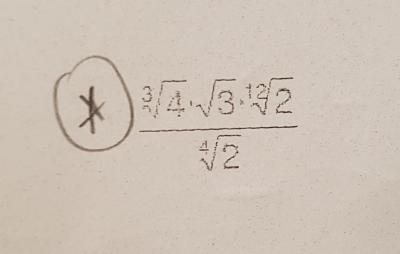Se muestran los artículos pertenecientes a Julio de 2018.

## Paola, Marta. Math classes July, 4ESO August 2018. Homework.

Publicado: 27/07/2018 19:17 por easyfisquim en Paola y Marta Math.27th July Friday class.

-  I remind you that these are the 5 exercises you have to do for the next class on 31st July Tuesday :
I  also post the results here for you to check if you get them or not.
( Let me know if you have any problem and write me then, have a nice weekend) .

- Simplify the following algebraic fractions :

- Rationalize the denominator :

- If you have extra time you could try to do the following one in order to remember how to expand a product :

------------------------------------------------------------------------------------------------------------------------------------------------

31st July Tuesday class.

Paola, Marta, at the following link you can look up, for the upcoming time, a bunch of exercises, just the kind of level you need, about simplifying, extanding, all kind of equations to solve, roots, logarithms, etc. If you have enough time, simply check this link and try to review some of the exercises starting at the bottom of the page and going to the top. You will find all sort of exercises, whose level is about the one you need to work. They are solved and provided with given solutions and explained step by step. If you have any problem just let me know it, anyway we will be working them out as we go furhter within the next lessons.

-  Homeworks corrections and solutions for the next class on thursday :

Homework solution 1               Homework solution 2

Homework solution 3               Homework solution 4

Homework solution 5               Homework solution 6

-----------------------------------------------------------------------------------------------------------------------------------------------

2nd August Thursday class.

Part 1 :  Reviewing quadratic functions and parabolas.

Link :       Website to draw parabolas.

-   The following videos will help you to understand how to transform a quadratic parabola function form in vertex from (video 1) , how to find the vertex of a parabola ( video 2 ) , how to find the equation of a parábola by completing the square ( video 3 )  and how to find the x and y intercept of a quadratic equation ( video 4 )  :

VIDEO 1                  VIDEO 2                   VIDEO 3                VIDEO 4

- To the following link you can find a website with a lot of logarithm equations solved, well explained and with a related YouTube video explained how to work them out step by step :

Logarithm and exponential equations.

--------------------------------------------------------------------------------------------------------------------

- Exemples done today in class about parabolas domain, range, x-intercepts, axis of symmetry and vertex .

Example 1  page 1                   Example 2  page 1                Example3    page1

1- Here you have, but corrected now, the exercise I did wrong :    Correction.

2- Homework to do for the next class on 7th Tuesday :

Homework 1                    Homework 2                    Homework 3

Homework 4                    Homework 5

-------------------------------------------------------------------------------------------------------------------------------------------

7th August Tuesday class.

- For what values of "b" will the following equation have no solution:  check and review

-------------------------------------------------------------------------------------------------------------------------------------------------

9th August Thursday class.

- Before checking the homework Im gonna post several videos for you to review main issues about absolute value equations and they related to absolute value inequations and graphing them :

----------------------------------------------------------------------------------------------------------------------------------------------------

14th August Tuesday class.

This homework1 is the one we did this morning in class. Its been posted here
only to help you remember the steps to be followed to achieve the problem.

- Homework 2 solved.   ( Piecewise function ).

- Homework 3 solved.   ( Piecewise function ).

- Homework 4 solved.   ( Piecewise function ).

- Homework 5 solved.    ( Exponential equations ).

- Homework 6 solved.    ( Inequations system drawing and range ).

----------------------------------------------------------------------------------------------------------------------------------------------------

16th August Thursday class.

----------------------------------------------------------------------------------------------------------------------------------------------------